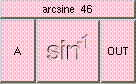ARCSINETHE BOX'S MAIN HELP: This function returns a scalar between [-PI/2,PI/2], the arcsine of A. Arcsine is the inverse of the sine function where the asin(x) = y if and only if sin(y) = x. A (input): This should be a scalar between [-1,1]. OUT (output): This function returns a scalar between [-PI/2,PI/2], the arcsine of A. Arcsine is the inverse of the sine function where the asin(x) = y if and only if sin(y) = x.

<-- BACK TO Math